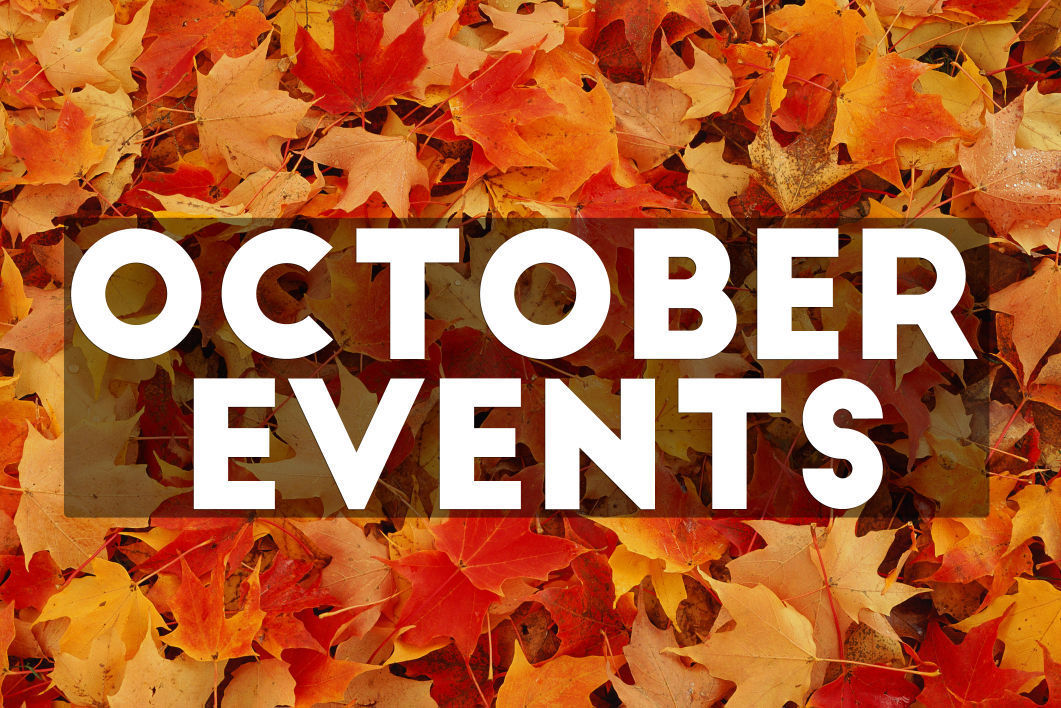# Important October Dates

Monday, October 12 - Indigenous People's Day (no school)
Wednesday, October 14 - PSAT/NMST Exam (in-person)
Thursday, October 15 - NCP Athletic Boosters Meeting (online)
Sunday, October 18 - Last day to order NCP gear
Tuesday, October 20 - LSC candidates forum (online)
Tuesday, October 27 - Friends of Northside meeting (online)

 table div table+table+table div table{width:100%;padding:0}table div table+table+table div table img{width:96.23%;padding:0;float:none}table div table+table+table div table td{width:100%;padding:0 1.88% 18px}/* styles */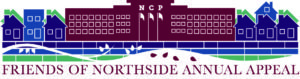table div table+table+table+table+table div table{width:100%;padding:0}table div table+table+table+table+table div table img{width:96.23%;padding:0;float:none}table div table+table+table+table+table div table td{width:100%;padding:0 1.88% 18px}/* styles */# Annual Appeal Letters Will Soon Be in the Mail

Please check your mailbox during the next couple of weeks for an important Annual Appeal letter from Principal Mest. It explains why annual giving is essential to maintaining Northside's excellent reputation and how Annual Appeal giving contributes directly to the educational experience of your child.

The mailing includes a form that outlines methods and levels of giving. Our parent volunteers have been working hard to ensure that your giving experience goes as smoothly as possible. You have many choices: check, money order, online payment with credit card, gift of stock shares or securities, monthly giving — we can handle it all!

 table div table+table+table+table+table+table+table div table{width:100%;padding:0}table div table+table+table+table+table+table+table div table img{width:96.23%;padding:0;float:none}table div table+table+table+table+table+table+table div table td{width:100%;padding:0 1.88% 18px}/* styles */# All NCP Communications Now Being Sent Via CNXT

NCP is now using SchoolCNXT to communicate important information to parents and the school community. All families should have received a text message and/or email communication about signing up.

If you did not receive that communication or need access to your student's account, you can gain access by using this tool on Northside's website.

 table div table+table+table+table+table+table+table+table+table+table div table{width:100%;padding:0}table div table+table+table+table+table+table+table+table+table+table div table img{width:96.23%;padding:0;float:none}table div table+table+table+table+table+table+table+table+table+table div table td{width:100%;padding:0 1.88% 18px}/* styles */# This Monday: No School - CPS Day Off

Monday, October 12
Indigenous People's Day/Columbus Day

 table div table+table+table+table+table+table+table+table+table+table+table+table div table{width:100%;padding:0}table div table+table+table+table+table+table+table+table+table+table+table+table div table img{width:96.23%;padding:0;float:none}table div table+table+table+table+table+table+table+table+table+table+table+table div table td{width:100%;padding:0 1.88% 18px}/* styles */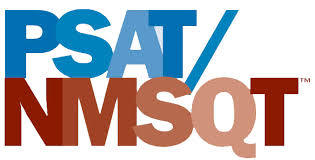# PSAT/NMST Exam

Wednesday, October 14
8:00 am

Northside College Prep juniors will participate in the PSAT/NMSQT exam. On the day of the exam, students are expected to report to school no later than 8:00 am for a full day of school.

 table div table+table+table+table+table+table+table+table+table+table+table+table+table+table div table{width:100%;padding:0}table div table+table+table+table+table+table+table+table+table+table+table+table+table+table div table img{width:96.23%;padding:0;float:none}table div table+table+table+table+table+table+table+table+table+table+table+table+table+table div table td{width:100%;padding:0 1.88% 18px}/* styles */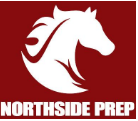# NCP Athletic Boosters Meeting

Thursday, October 15
7:00 pm
Zoom Meeting

The Athletic Boosters Committee will be hosting their second virtual meeting of the school year on Thursday, October 15 at 7:00 pm. All NCP parents are welcome to attend. If interested, please email Mike Hughes at
mhughes@nge.com for details. You can use the following link or meeting information to join via Zoom.

Meeting ID: 988 710 6172
Passcode: 389788

Go Mustangs!

 table div table+table+table+table+table+table+table+table+table+table+table+table+table+table+table+table div table{width:100%;padding:0}table div table+table+table+table+table+table+table+table+table+table+table+table+table+table+table+table div table img{width:96.23%;padding:0;float:none}table div table+table+table+table+table+table+table+table+table+table+table+table+table+table+table+table div table td{width:100%;padding:0 1.88% 18px}/* styles */# LSC Virtual Candidate Forum

Tuesday, October 20
6:00 pm
Zoom Meeting

A Virtual Forum for the 2020 Local School Council Election will be held at 6:00 pm on Tuesday, October 20. We encourage and invite you to attend the Forum to hear the Candidates speak about their qualifications and
their plans if elected (or appointed) to Northside's LSC.

To join the Zoom Meeting, click HERE or call 1-312-626-6799 and enter PIN ​095140​.

 table div table+table+table+table+table+table+table+table+table+table+table+table+table+table+table+table+table+table div table{width:100%;padding:0}table div table+table+table+table+table+table+table+table+table+table+table+table+table+table+table+table+table+table div table img{width:96.23%;padding:0;float:none}table div table+table+table+table+table+table+table+table+table+table+table+table+table+table+table+table+table+table div table td{width:100%;padding:0 1.88% 18px}/* styles */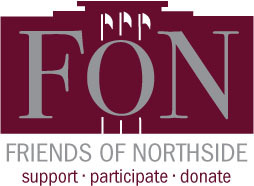# Friends of Northside

Tuesday, October 27
7:00 pm

Please join Friends of Northside for their upcoming meeting.

 table div table+table+table+table+table+table+table+table+table+table+table+table+table+table+table+table+table+table+table+table div table{width:100%;padding:0}table div table+table+table+table+table+table+table+table+table+table+table+table+table+table+table+table+table+table+table+table div table img{width:96.23%;padding:0;float:none}table div table+table+table+table+table+table+table+table+table+table+table+table+table+table+table+table+table+table+table+table div table td{width:100%;padding:0 1.88% 18px}/* styles */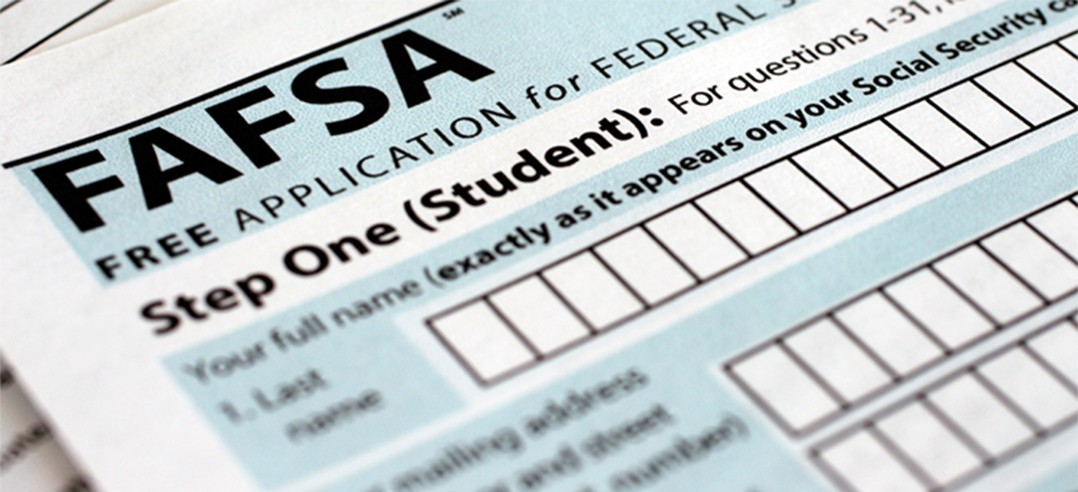## Financial Aid Resources

The FAFSA Application, RISE Application for undocumented and transgender students, and the College Board: College Scholarship Service Profile (CSS Profile) are now open! The class of 2021 parents and students can begin to submit their respective financial aid applications. Please review the information below for resources to assist your family with navigating the financial aid process.

## Virtual Financial Aid Presentations

▪ UChicago Financial Aid Session (click here) - Monday, October 19, 2020, at 11:00 am until 12:00 pm
▪ Illinois State University Virtual Financial Aid Session (click here) - Thursday, November 12, 2020, at 12:00 pm
 ▪ UChicago Financial Aid Session (click here) - Monday, October 19, 2020, at 11:00 am until 12:00 pm
 ▪ Illinois State University Virtual Financial Aid Session (click here) - Thursday, November 12, 2020, at 12:00 pm

## NCP Virtual Financial Aid Workshops

Northside Prep Counseling Department will offer daytime and evening financial aid workshops for students and parents. Click here to register for the upcoming financial aid workshops. The financial aid workshops will offer students and parents an opportunity to receive support with completing the FAFSA Application, RISE Application for undocumented and transgender students, and the College Board: College Scholarship Service Profile (CSS Profile). Please note, the financial aid workshops are recommended for the class of 2021 students and parents only.

▪ Wednesday, October 14 (6:00 pm until 8:00 pm) -- English & Spanish
▪ Wednesday, October 21 (1:25 pm until 2:10 pm)
▪ Wednesday, October 21 (2:15 pm until 3:00 pm)
▪ Wednesday, October 21 (6:00 pm until 8:00 pm)
▪ Thursday, November 5 (1:25 pm until 2:10 pm)
▪ Thursday, November 5 (2:15 pm until 3:00 pm)
 ▪ Wednesday, October 14 (6:00 pm until 8:00 pm) -- English & Spanish
 ▪ Wednesday, October 21 (1:25 pm until 2:10 pm)
 ▪ Wednesday, October 21 (2:15 pm until 3:00 pm)
 ▪ Wednesday, October 21 (6:00 pm until 8:00 pm)
 ▪ Thursday, November 5 (1:25 pm until 2:10 pm)
 ▪ Thursday, November 5 (2:15 pm until 3:00 pm)
 table div table+table+table+table+table+table+table+table+table+table+table+table+table+table+table+table+table+table+table+table+table+table+table div table{width:100%;padding:0}table div table+table+table+table+table+table+table+table+table+table+table+table+table+table+table+table+table+table+table+table+table+table+table div table img{width:96.23%;padding:0;float:none}table div table+table+table+table+table+table+table+table+table+table+table+table+table+table+table+table+table+table+table+table+table+table+table div table td{width:100%;padding:0 1.88% 18px}/* styles */# Photo Submissions Requested for NCP Stampede!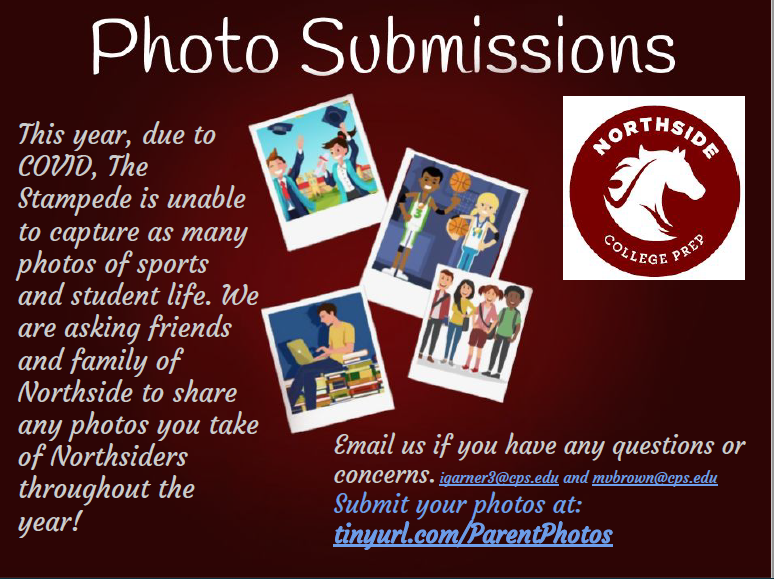table div table+table+table+table+table+table+table+table+table+table+table+table+table+table+table+table+table+table+table+table+table+table+table+table+table+table div table{width:100%;padding:0}table div table+table+table+table+table+table+table+table+table+table+table+table+table+table+table+table+table+table+table+table+table+table+table+table+table+table div table img{width:96.23%;padding:0;float:none}table div table+table+table+table+table+table+table+table+table+table+table+table+table+table+table+table+table+table+table+table+table+table+table+table+table+table div table td{width:100%;padding:0 1.88% 18px}/* styles */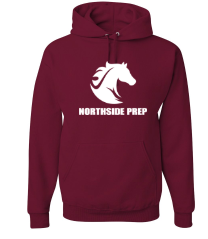Buy NCP spirit wear HERE! The Saddle Shop is open through Sunday, October 18. Each item ordered will be custom produced, so there are no returns or exchanges.

Please note, orders will be shipped 15 business days after the store closes.

 table div table+table+table+table+table+table+table+table+table+table+table+table+table+table+table+table+table+table+table+table+table+table+table+table+table+table+table+table div table{width:100%;padding:0}table div table+table+table+table+table+table+table+table+table+table+table+table+table+table+table+table+table+table+table+table+table+table+table+table+table+table+table+table div table img{width:96.23%;padding:0;float:none}table div table+table+table+table+table+table+table+table+table+table+table+table+table+table+table+table+table+table+table+table+table+table+table+table+table+table+table+table div table td{width:100%;padding:0 1.88% 18px}/* styles */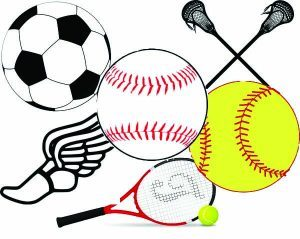# This Week in Sports

Come out and support our student-athletes!

## Women's Swimming

▪ Wednesday, October 14, TBD (V All-City) vs. Chicago Public at NCP
 ▪ Wednesday, October 14, TBD (V All-City) vs. Chicago Public at NCP

## Women's Cross Country

▪ Monday, October 12, TBD (V All-City CPS Open Championships) vs. Chicago Public at Washington Park
 ▪ Monday, October 12, TBD (V All-City CPS Open Championships) vs. Chicago Public at Washington Park

## Men's Cross Country

▪ Monday, October 12, TBD (V All-City) vs. Chicago Public at Washington Park
 ▪ Monday, October 12, TBD (V All-City) vs. Chicago Public at Washington Park

## Women's Tennis

▪ Friday, October 16, TBD (V IHSA Sectional) vs. New Trier at New Trier High School
 ▪ Friday, October 16, TBD (V IHSA Sectional) vs. New Trier at New Trier High School

 table div table+table+table+table+table+table+table+table+table+table+table+table+table+table+table+table+table+table+table+table+table+table+table+table+table+table+table+table+table+table div table{width:100%;padding:0}table div table+table+table+table+table+table+table+table+table+table+table+table+table+table+table+table+table+table+table+table+table+table+table+table+table+table+table+table+table+table div table img{width:96.23%;padding:0;float:none}table div table+table+table+table+table+table+table+table+table+table+table+table+table+table+table+table+table+table+table+table+table+table+table+table+table+table+table+table+table+table div table td{width:100%;padding:0 1.88% 18px}/* styles */# Do you have news or team results to share with the Northside parent community?

 table div table+table+table+table+table+table+table+table+table+table+table+table+table+table+table+table+table+table+table+table+table+table+table+table+table+table+table+table+table+table+table+table+table div table{width:100%;padding:0}table div table+table+table+table+table+table+table+table+table+table+table+table+table+table+table+table+table+table+table+table+table+table+table+table+table+table+table+table+table+table+table+table+table div table img{width:96.23%;padding:0;float:none}table div table+table+table+table+table+table+table+table+table+table+table+table+table+table+table+table+table+table+table+table+table+table+table+table+table+table+table+table+table+table+table+table+table div table td{width:100%;padding:0 1.88% 18px}/* styles */# Support Northside - Shop AmazonSmile

AmazonSmile is a simple and automatic way to support Northside every time you shop.

Please note that our AmazonSmile name is Friends of "North" "Side" (North Side - two words at AmazonSmile).

 table div table+table+table+table+table+table+table+table+table+table+table+table+table+table+table+table+table+table+table+table+table+table+table+table+table+table+table+table+table+table+table+table+table+table+table div table{width:100%;padding:0}table div table+table+table+table+table+table+table+table+table+table+table+table+table+table+table+table+table+table+table+table+table+table+table+table+table+table+table+table+table+table+table+table+table+table+table div table img{width:96.23%;padding:0;float:none}table div table+table+table+table+table+table+table+table+table+table+table+table+table+table+table+table+table+table+table+table+table+table+table+table+table+table+table+table+table+table+table+table+table+table+table div table td{width:100%;padding:0 1.88% 18px}/* styles */# Follow Us on Social Media

 table div table+table+table+table+table+table+table+table+table+table+table+table+table+table+table+table+table+table+table+table+table+table+table+table+table+table+table+table+table+table+table+table+table+table+table+table+table div table{width:100%;padding:0}table div table+table+table+table+table+table+table+table+table+table+table+table+table+table+table+table+table+table+table+table+table+table+table+table+table+table+table+table+table+table+table+table+table+table+table+table+table div table img{width:96.23%;padding:0;float:none}table div table+table+table+table+table+table+table+table+table+table+table+table+table+table+table+table+table+table+table+table+table+table+table+table+table+table+table+table+table+table+table+table+table+table+table+table+table div table td{width:100%;padding:0 1.88% 18px}/* styles */table div table+table+table+table+table+table+table+table+table+table+table+table+table+table+table+table+table+table+table+table+table+table+table+table+table+table+table+table+table+table+table+table+table+table+table+table+table+table+table div table{width:100%;padding:0}table div table+table+table+table+table+table+table+table+table+table+table+table+table+table+table+table+table+table+table+table+table+table+table+table+table+table+table+table+table+table+table+table+table+table+table+table+table+table+table div table img{width:96.23%;padding:0;float:none}table div table+table+table+table+table+table+table+table+table+table+table+table+table+table+table+table+table+table+table+table+table+table+table+table+table+table+table+table+table+table+table+table+table+table+table+table+table+table+table div table td{width:100%;padding:0 1.88% 18px}/* styles */table div table+table+table+table+table+table+table+table+table+table+table+table+table+table+table+table+table+table+table+table+table+table+table+table+table+table+table+table+table+table+table+table+table+table+table+table+table+table+table+table+table+table div table{width:100%;padding:0}table div table+table+table+table+table+table+table+table+table+table+table+table+table+table+table+table+table+table+table+table+table+table+table+table+table+table+table+table+table+table+table+table+table+table+table+table+table+table+table+table+table+table div table img{width:96.23%;padding:0;float:none}table div table+table+table+table+table+table+table+table+table+table+table+table+table+table+table+table+table+table+table+table+table+table+table+table+table+table+table+table+table+table+table+table+table+table+table+table+table+table+table+table+table+table div table td{width:100%;padding:0 1.88% 18px}/* styles */